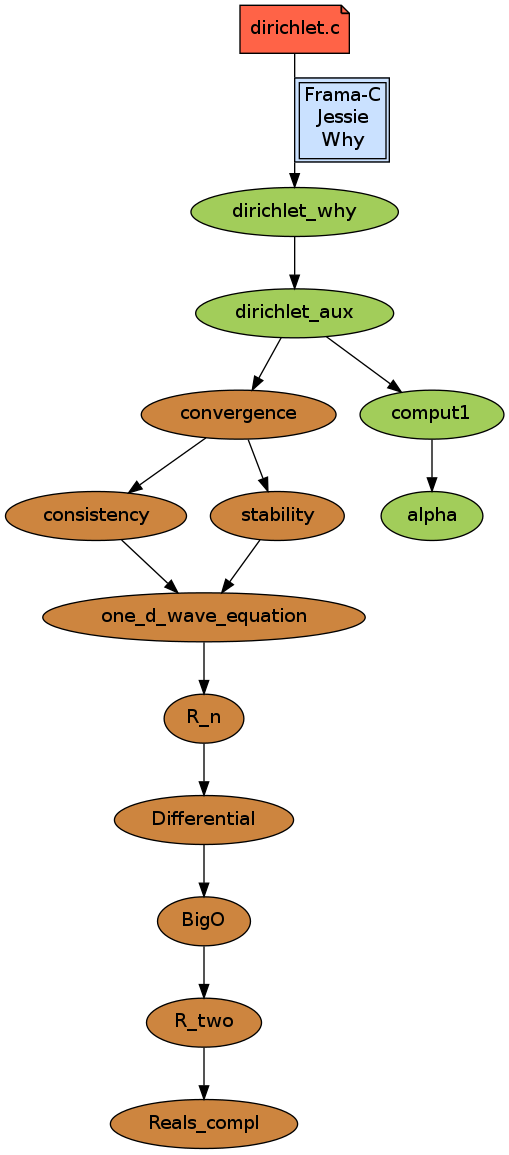## Rounding and method errors of a numerical scheme for the wave equation

The annotated C file and the Coq proofs are available here as .tar.gz.

You may also browse the files:Here is the list of all the generated verification conditions (VC) and how thery are proved. The Coq proofs (before the VC and for the VC) are also cited, with their size and compilation times.

 Proof obligations Alt-Ergo CVC3 Gappa Z3 Coq Previous Coq proof about convergence 4108 59.71 Previous Coq proof about FP error 1623 1min 07 Theory VC Theory realization 80 1. Lemma alpha_conv_pos 17 2. Lemma analytic_error_le 244 3. sqr_norm_dx_conv_err_0 5 4. sqr_norm_dx_conv_err_succ 12 Other lemmas about realization 1595 Total for VC realization & lemmas 1953 20 min 18 Behavior VC 1. assertion 2. assertion 3. assertion 113 4. assertion 5 5. assertion 35 6. assertion 14 7. assertion 26 8. assertion 40 9. loop invariant initially holds 10. loop invariant initially holds 11. loop invariant initially holds 4 12. loop invariant preserved 13. loop invariant preserved 14. loop invariant preserved 22 15. assertion 6 16. loop invariant initially holds 17. loop invariant initially holds 18. loop invariant initially holds 6 19. assertion 20. assertion 21. assertion 22. loop invariant preserved 23. loop invariant preserved 24. loop invariant preserved 273 25. assertion 6 26. loop invariant initially holds 27. loop invariant initially holds 28. loop invariant initially holds 29. loop invariant initially holds 30. loop invariant initially holds 31. loop invariant initially holds 5 32. assertion 3 33. assertion 3 34. assertion 3 35. assertion 3 36. loop invariant preserved 37. loop invariant preserved 38. loop invariant preserved 238 39. assertion 6 40. loop invariant preserved 41. loop invariant preserved 42. loop invariant preserved 43. postcondition 19 44. postcondition 115 Safety VC 1. floating-point overflow 2. floating-point overflow 3. floating-point overflow 4. floating-point overflow 5. floating-point overflow 71 6. floating-point overflow 48 7. floating-point overflow 46 8. arithmetic overflow 9. arithmetic overflow 10. arithmetic overflow 11. arithmetic overflow 12. precondition for user call 13. precondition for user call 14. pointer dereferencing 15. pointer dereferencing 16. pointer dereferencing 17. pointer dereferencing 18. floating-point overflow 12 19. floating-point overflow 20. precondition for user call 21. precondition for user call 213 22. pointer dereferencing 23. pointer dereferencing 24. pointer dereferencing 25. pointer dereferencing 26. arithmetic overflow 27. arithmetic overflow 28. variant decreases 29. variant decreases 30. pointer dereferencing 31. pointer dereferencing 32. pointer dereferencing 33. pointer dereferencing 34. pointer dereferencing 35. pointer dereferencing 36. arithmetic overflow 37. arithmetic overflow 38. pointer dereferencing 39. pointer dereferencing 40. pointer dereferencing 41. pointer dereferencing 42. pointer dereferencing 43. pointer dereferencing 44. pointer dereferencing 45. pointer dereferencing 46. floating-point overflow 47. floating-point overflow 6 48. arithmetic overflow 49. arithmetic overflow 50. pointer dereferencing 51. pointer dereferencing 52. pointer dereferencing 53. pointer dereferencing 54. floating-point overflow 7 55. pointer dereferencing 56. pointer dereferencing 57. floating-point overflow 58. floating-point overflow 59. floating-point overflow 14 60. pointer dereferencing 61. pointer dereferencing 62. variant decreases 63. variant decreases 64. pointer dereferencing 65. pointer dereferencing 66. arithmetic overflow 67. arithmetic overflow 68. pointer dereferencing 69. pointer dereferencing 70. arithmetic overflow 71. arithmetic overflow 72. pointer dereferencing 73. pointer dereferencing 74. pointer dereferencing 75. pointer dereferencing 76. pointer dereferencing 77. pointer dereferencing 78. pointer dereferencing 79. pointer dereferencing 80. floating-point overflow 81. floating-point overflow 8 82. arithmetic overflow 83. arithmetic overflow 84. pointer dereferencing 85. pointer dereferencing 86. pointer dereferencing 87. pointer dereferencing 88. floating-point overflow 7 89. pointer dereferencing 90. pointer dereferencing 91. floating-point overflow 92. arithmetic overflow 93. arithmetic overflow 94. pointer dereferencing 95. pointer dereferencing 96. floating-point overflow 8 97. floating-point overflow 98. floating-point overflow 15 99. precondition 100. precondition 101. variant decreases 102. variant decreases 103. pointer dereferencing 104. pointer dereferencing 105. variant decreases 106. variant decreases Total for behavior and safety VCs 1400 11 min 56